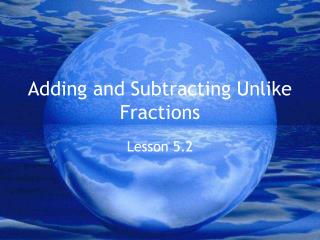DownloadDownload Presentation# Adding and Subtracting Unlike Fractions

Télécharger la présentation## Adding and Subtracting Unlike Fractions

- - - - - - - - - - - - - - - - - - - - - - - - - - - E N D - - - - - - - - - - - - - - - - - - - - - - - - - - -
##### Presentation Transcript

1. Adding and Subtracting Unlike Fractions Lesson 5.2

2. Reading a Diagram • If the diagram has two rows that are even, it is an addition problem. • The addition problem is found in the top row. • The answer is found in the bottom row.

3. Example The top row is “one half plus one fourth” 1 2 1 4 The bottom row holds the answer: Count up the fourths. We have three fourths. 1 4 1 4 1 4

4. Subtracting With a Diagram • If the top row and bottom row is uneven, then it is a subtraction problem. • Think of it as the top row minus the bottom row.

5. Example 1 12 1 12 1 12 1 12 1 12 1 4 This problem says: 5/12 - 1/4 Cancel out the one fourth, and the same amount on the top row. Count what is left: two twelfths. When you reduce 2/12, you get 1/6.

6. Most problems will be solved with pencil and paper. • List the multiples of both denominators. • Find the least common multiple (LCM). • Write new fractions with the LCM as the new denominator. • Find the factor you multiply by to get from your original denominator to your new denominator. • Use that same factor, and multiply it by your original numerator to get a new numerator.

7. WHAT DOES THAT MEAN? Let’s illustrate the steps with an example. 3 1 + 4 6

8. 3 1 + 4 6 9 2 x 3 x 2 + 12 12 11 Multiples of 4: 4, 8, 12, 16, 20 12 Multiples of 6: 6, 12, 18, 24, 30

9. Example 2 9 2 10, 20, 30, 40, 50 5, 10, 15, 20, 25 5 10 5 x 1 x 2 9 4 = 10 10 10 1 = 2

10. Homework Time!